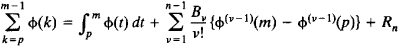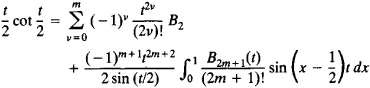# Euler-Maclaurin Summation Formula

The following article is from The Great Soviet Encyclopedia (1979). It might be outdated or ideologically biased.

## Euler-Maclaurin Summation Formula

a formula relating the partial sums of an infinite series to the integral and derivatives of the general term of the series:where the Bv are Bernoulli numbers and Rn is the remainder.

The formula is used for the approximate computation of definite integrals, for the investigation of the convergence of infinite series, for the computation of sums, and for the expansion of functions in Taylor’s series. For example, if m = 1, p = 0, n = 2m + 1, and φ(x) = cos (xt/2), the formula gives the following expression:First introduced by L. Euler in 1738, the formula was discovered independently by C. Maclaurin in 1742.